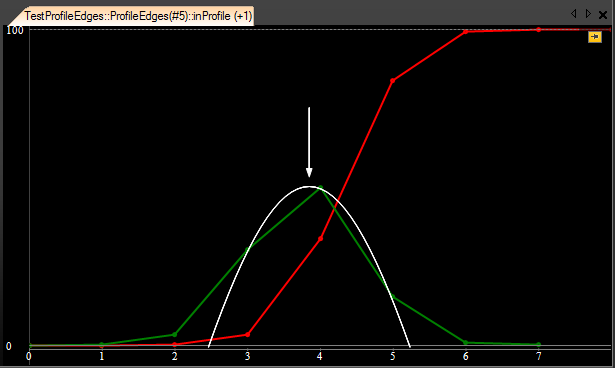You are here: Start » 1D Edge Detection – Subpixel Precision

# 1D Edge Detection – Subpixel Precision

## Introduction

One of the key strengths of the 1D Edge Detection tools is their ability do detect edges with precision higher than the pixel grid. This is possible, because the values of the derivative profile (of pixel values) can be interpolated and its maxima can be found analytically.

## Example: Parabola Fitting

Let us consider a sample profile of pixel values corresponding to an edge (red):Sample edge profile (red) and its derivative (green). Please note, that the derivative is shifted by 0.5.

The steepest segment is between points 4.0 and 5.0, which corresponds to the maximum of the derivative (green) at 4.5. Without the subpixel precision the edge would be found at this point.

It is, however, possible to consider information about the values of the neighbouring profile points to extract the edge location with higher precision. The simplest method is to fit a parabola to three consecutive points of the derivative profile:Fitting a parabola to three consecutive points.

Now, the edge point we are looking for can be taken from the maximum of the parabola. In this case it will be 4.363, which is already a subpixel-precise result. This precision is still not very high, however. We know it from an experiment – this particular profile, which we are considering in this example, has been created from a perfect gaussian edge located at the point 430 and downsampled 100 times to simulate a camera looking at an edge at the point 4.3. The error that we got, is 0.063 px. From other experiments we know that in the worst case it can be up to 1/6 px.

## Advanced: Methods Available in Aurora Vision

More advanced methods can be used that consider not three, but four consecutive points and which employ additional techniques to assure the highest precision in presence of noise and other practical edge distortions. In Aurora Vision Studio they are available in a form of 3 different profile interpolation methods:

• Linear – the simplest method that results in pixel-precise results,
• Quadratic3 – an improved fitting of parabola to 3 consecutive points,
• Quadratic4 – an advanced method that fits parabola to 4 consecutive points.

The precision of these methods on perfect gaussian edges is respectively: 1/2 px, 1/6 px and 1/23 px. It has to be added, however, that the Quadratic4 method differs significantly in its performance on edges which are only slightly blurred – when the image quality is close to perfect, the precision can be even higher than 1/50 px.

 Previous: 1D Edge Detection Next: Shape Fitting